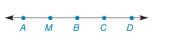Chapter 2.2, Problem 26E### Elementary Geometry for College St...

6th Edition
Daniel C. Alexander + 1 other
ISBN: 9781285195698

#### Solutions

Chapter
Section### Elementary Geometry for College St...

6th Edition
Daniel C. Alexander + 1 other
ISBN: 9781285195698
Textbook Problem
1 views

# In Exercises 23 to 35, give the indirect proof for each problem or statement. Given: M B > B C A M = C D Prove: B is not themidpoint of A D ¯To determine

To prove:

The given statement.

Explanation

Given:

The given statement is,

MB>BC and AM=CD

Figure (1)

Property:

A point B lies between the points A and C of line segment AC if and only if, AB+BC=AC.

Approach:

Assume that B is the midpoint of AD¯.

AB¯=BD¯

Point M lies between the points A and B. Point C lies between the points B and D in figure (1).

By above stated property,

AB¯=AM¯+MB¯BD¯=BC¯+CD¯AM¯+MB¯=BC¯+CD¯

### Still sussing out bartleby?

Check out a sample textbook solution.

See a sample solution

#### The Solution to Your Study Problems

Bartleby provides explanations to thousands of textbook problems written by our experts, many with advanced degrees!

Get Started